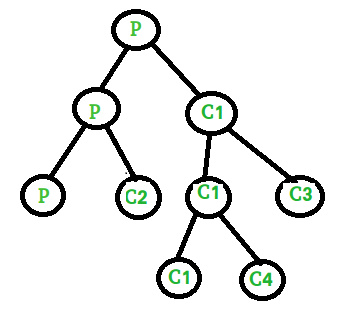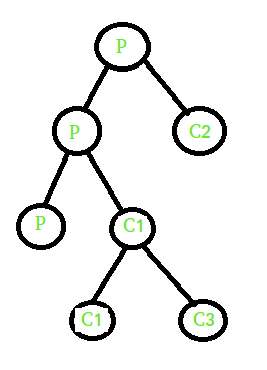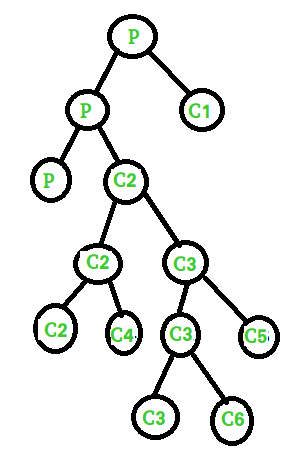# Fork() – Practice questions

• Difficulty Level : Medium
• Last Updated : 17 Feb, 2020

Prerequisite: basics of fork, fork and binary tree,

Example1:
What is the output of the following code?

 `#include ``#include ``int` `main()``{``    ``if` `(fork() || fork())``        ``fork();``    ``printf``(``"1 "``);``    ``return` `0;``}`

Output:

` 1 1 1 1 1 `Explanation:
1. It will create two process one parent P (has process ID of child process)and other is child C1 (process ID = 0).
2. In if statement we used OR operator( || ) and in this case second condition is evaluated when first condition is false.
3. Parent process P will return positive integer so it directly execute statement and create two more processes (one parent P and other is child C2). Child process C1 will return 0 so it checks for second condition and second condition again create two more processes(one parent C1 and other is child C3).
4. C1 return positive integer so it will further create two more processes (one parent C1 and other is child C4). Child C3 return 0 so it will directly print 1.

Example 2:
What is the output of following code?

 `#include ``#include `` ` `int` `main()``{``    ``if` `(fork()) {``        ``if` `(!fork()) {``            ``fork();``            ``printf``(``"1 "``);``        ``}``        ``else` `{``            ``printf``(``"2 "``);``        ``}``    ``}``    ``else` `{``        ``printf``(``"3 "``);``    ``}``    ``printf``(``"4 "``);``    ``return` `0;``}`

Output:

` 2 4 1 4 1 4 3 4 `Explanation:
1. It will create two process one parent P (has process ID of child process) and other is child C1 (process ID = 0).
2. When condition is true parent P executes if statement and child C1 executes else statement and print 3. Parent P checks next if statement and create two process (one parent P and child C2). In if statement we are using not operator (i.e, !), it executes for child process C2 and parent P executes else part and print value 2. Child C2
further creates two new processes (one parent C2 and other is child C3).

Example 3:
What is the output of following code?

 `#include ``#include `` ` `int` `main()``{``    ``if` `(fork() && (!fork())) {``        ``if` `(fork() || fork()) {``            ``fork();``        ``}``    ``}``    ``printf``(``"2 "``);``    ``return` `0;``}`

Output:

` 2 2 2 2 2 2 2 `Explanation:
1. Fork will create two process one parent P (has process id of new child) and other one is child C1 (process id=0).
2. In if statement we are using AND operator (i.e, &&) and in this case if first condition is false then it will not evaluate second condition and print 2. Parent process P check for second condition and create two new processes (one parent P and other is child C2). In second condition we are using NOT operator which return true for child process C2 and it executes inner if statement.
3. Child C2 again create two new processes (one parent C2 and child C3) and we are using OR operator (i.e, ||) which evaluate second condition when first condition is false. Parent C2 execute if part and create two new processes (one parent C2 and child C4) whereas child C3 check for second condition and create two new processes (one parent C3 and child C5).
4. Parent C3 enters in if part and further create two new processes (one parent C3 and child C6).

My Personal Notes arrow_drop_up